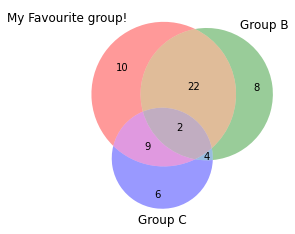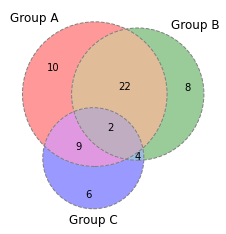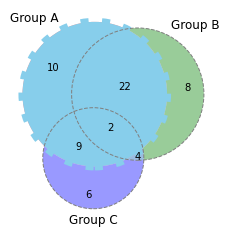# Custom Venn diagram

The posts #170 and #171 show how to make 2 and 3 groups Venn diagrams respectively. This page aims to give a few keys to custom these graphics.

## Custom Label

You can custom the label of a specific group. In the example below, the label is selected by id using the `get_label_by_id()` function and then customized by the function `set_text()`.

``````# Import the library
import matplotlib.pyplot as plt
from matplotlib_venn import venn3

# Custom text labels: change the label of group A
v=venn3(subsets = (10, 8, 22, 6,9,4,2), set_labels = ('Group A', 'Group B', 'Group C'))
v.get_label_by_id('A').set_text('My Favourite group!')
plt.show()``````## Line Style of Circles

It is possible to change the line style of circles using the `venn3_circles()` function.

``````# Import the library
import matplotlib.pyplot as plt
from matplotlib_venn import venn3, venn3_circles

# Line style: can be 'dashed' or 'dotted' for example
v=venn3(subsets = (10, 8, 22, 6,9,4,2), set_labels = ('Group A', 'Group B', 'Group C'))
c=venn3_circles(subsets = (10, 8, 22, 6,9,4,2), linestyle='dashed', linewidth=1, color="grey")
plt.show()``````## Custom One Group Only

After creating the circles by `venn3_circles()`, you can select the group you want to customize by its id and use `set_lw()`, `set_ls()`, and `set_color()` functions to make customizations.

``````# Import the library
import matplotlib.pyplot as plt
from matplotlib_venn import venn3, venn3_circles

# Change one group only
v=venn3(subsets = (10, 8, 22, 6,9,4,2), set_labels = ('Group A', 'Group B', 'Group C'))
c=venn3_circles(subsets = (10, 8, 22, 6,9,4,2), linestyle='dashed', linewidth=1, color="grey")
c.set_lw(8.0)
c.set_ls('dotted')
c.set_color('skyblue')
plt.show()``````## Contact & Edit

👋 This document is a work by Yan Holtz. Any feedback is highly encouraged. You can fill an issue on Github, drop me a message onTwitter, or send an email pasting `yan.holtz.data` with `gmail.com`.